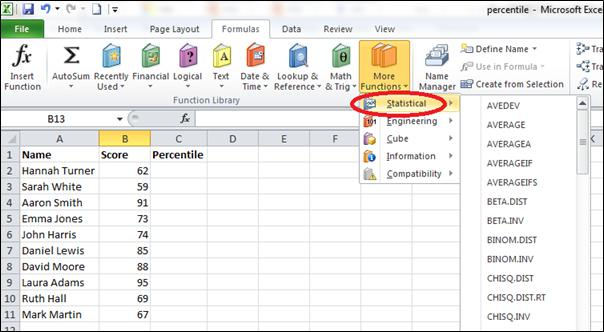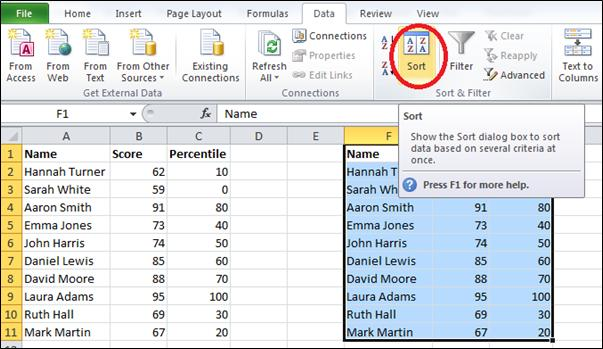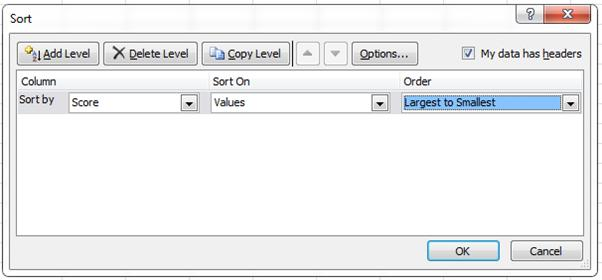### How to Calculate Percentile in Excel

If you have written any competitive exams, you might be familiar with the term percentile. Most of today’s competitive entrance exams publish the results of the applicants based on percentiles. You might be familiar with the term percent. But, it is not the same as percentile. Do not confuse these terms. As we all know, percentage is a number defined as a fraction of 100. So, if a person says that he scored 60% marks in his GMAT exam, then it means that if total score is 100, he scored 60 marks. Obviously, if the total score is 50, the person has got 30 marks in the exam.

A percentile is a measure at or below which a certain percentage of the distribution lies. So, if a person says he scored 60 percentile in his GMAT exam, then it means that 60 percent of students scored equal to or lower than him and 40 percent of students exceeded his score. With this, you cannot tell that the person scored 60 marks in a 100 marks exam. Only information you get is that there are 59 students who scored less than your score and 40 students who scored more than you if the total number of students are 100. In short, percentage gives us correct information about how an individual performed on a test whereas with percentile, you cannot understand the actual score a person achieved. Percentile gives us information about how that score compares to the scores of other test takers.

Excel offers mainly four functions named PERCENTILE.INC, PERCENTILE.EXC, PERCENTRANK.INC and PERCENTRANK.EXC. Let’s calculate the relative percentile scores of 10 students. In that case, we can use either PERCENTRANK.INC or PERCENTRANK.EXC. The PERCENTRANK.INC function will return the result as a number between 0 and 1 (both inclusive) and PERCENTRANK.EXC function will return the result as a number between 0 and 1 (both exclusive).

# Calculate Percentile

Open Excel and save the file as percentile.xlsx. Type “Name”, “Score” and “Percentile” in the cells A1, A2 and A3. You can format these cells to make them bold. Type any ten names in cells from A2 to A11 and enter any ten marks in cells from B2 to B11. Now your screen will look like this (names and scores could be different):Click cell C2 and go to Formulas (main menu) –> More Functions (in the Function Library group) –> Statistical and select PERCENTRANK.INC function.You will get a window like this:In the textbox next to Array, enter “B\$2:B\$11” (without double quotes). Enter B2 in the textbox next to X and enter 1 in the textbox next to Significance. Now your window will look like this:Click OK. In the formula bar, enter “*100” (without double quotes) next to the formula.Now copy the formula in cell C1 and paste the same in cells C2 to C11. Now your screen will look like this:If you analyze the data, you could find that the percentile of Sarah White is 0 and the percentile of Laura Adams is 100. If you analyze the scores of all the ten students, you can see that the Sarah White has got the lowest score and Laura Adams has got the highest score. The percentile of John Harris is 50, which means that 50% of the students (5 out of 10 students) have scored less than John Harris. The students who scored less than John Harris are Hannah Turner, Sarah White, Emma Jones, Ruth Hall and Mark Martin (total 5 students).

If you sort the data by scores, you will get a clearer picture. Copy the whole data (cells A1 through C11) and paste it in cells from F1 through H11 in the same worksheet. Now your screen will look like this:Select the newly copied data and go to Data (main menu) –> Sort (in the Sort & Filter) section and click Sort.You will get a window like this:From the Sort by list, select Score and from the Order list, select Largest to Smallest. Now your screen will look like this:Click OK and now your data will be like this:Now you can analyze the data easily.

## Template

```Further reading: